# Physics 504 chapter 1 & 2 opticks

5 de May de 2014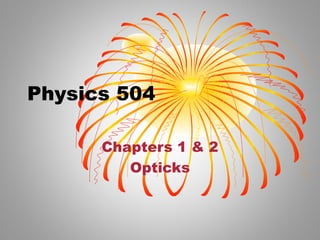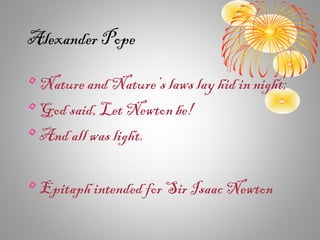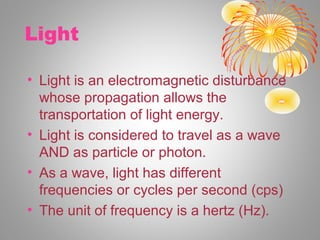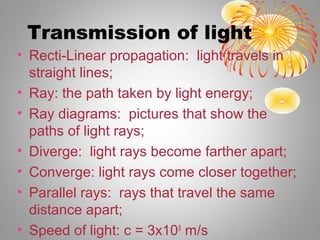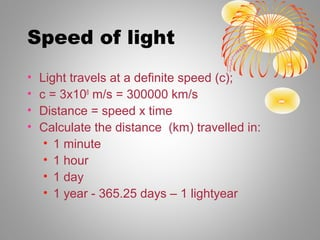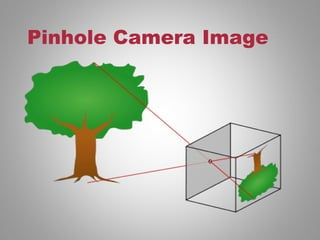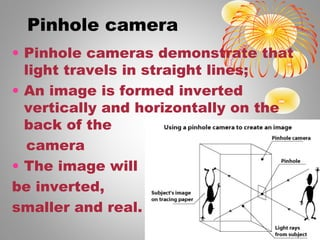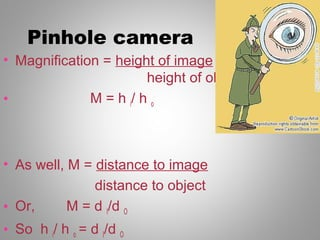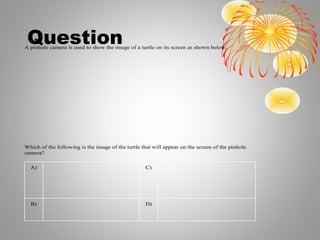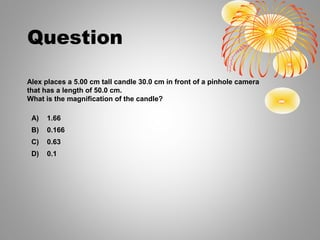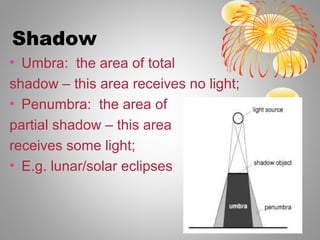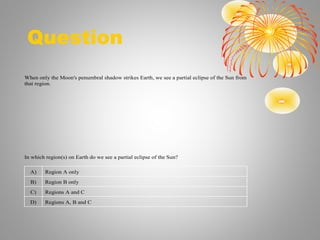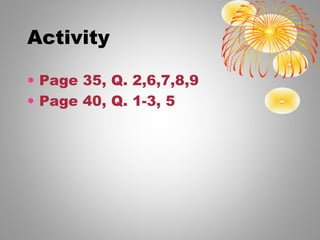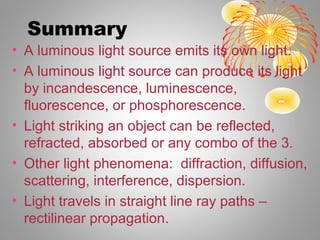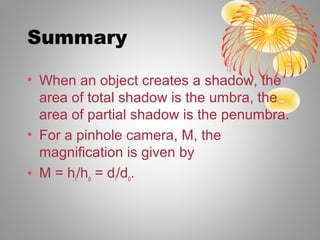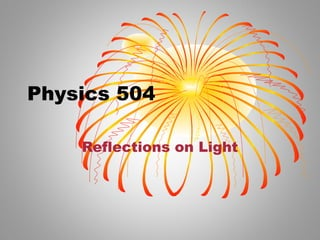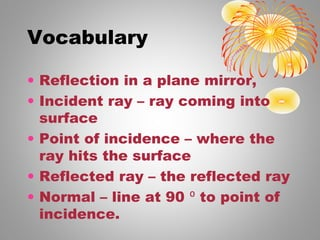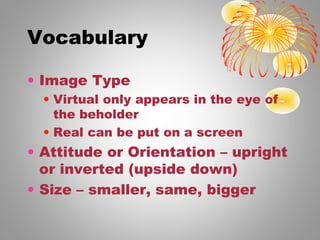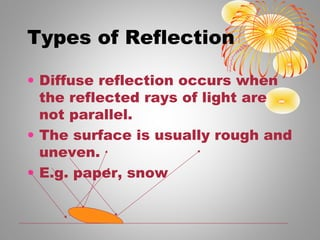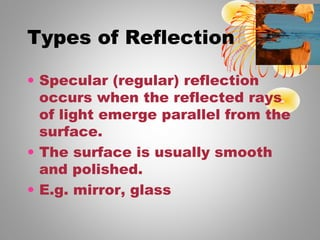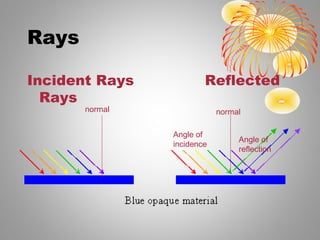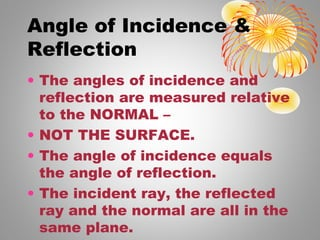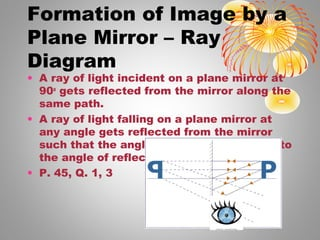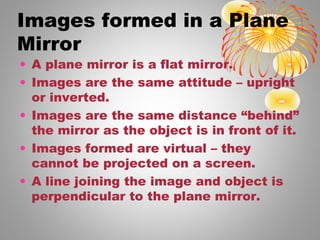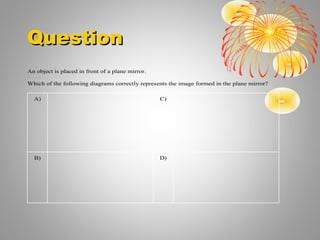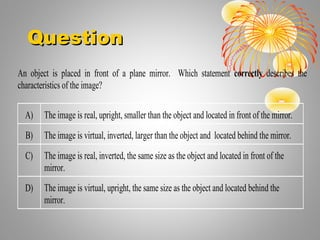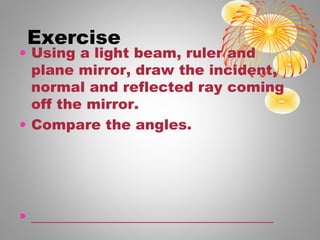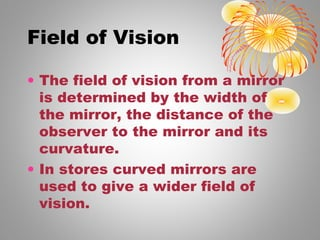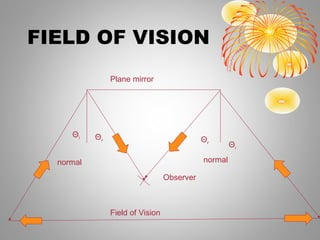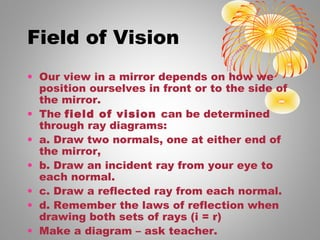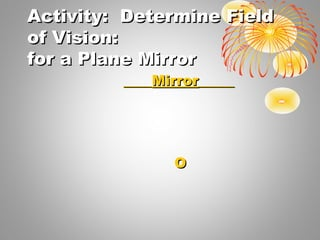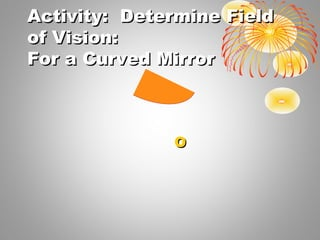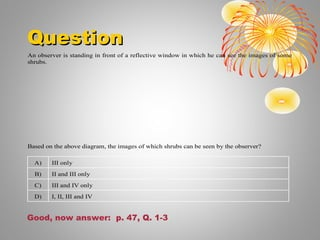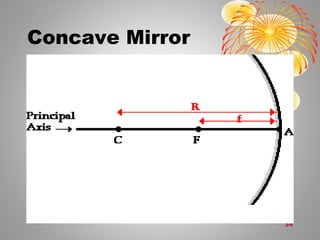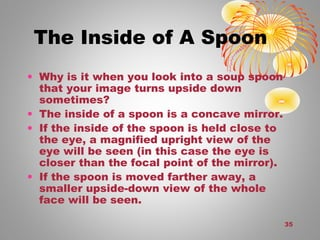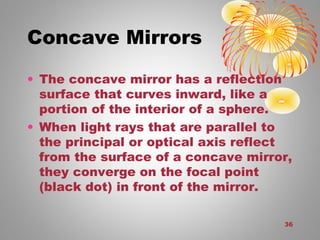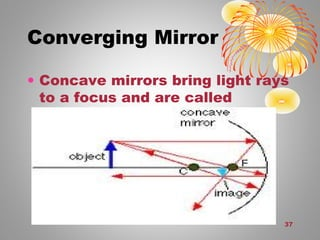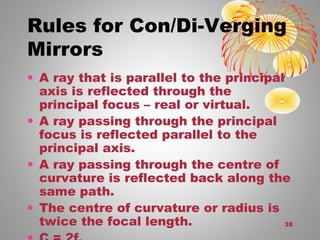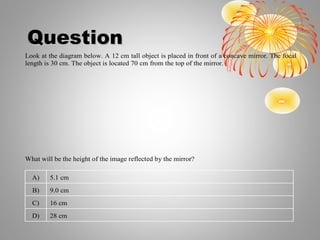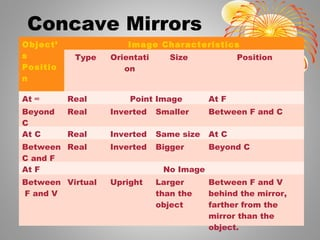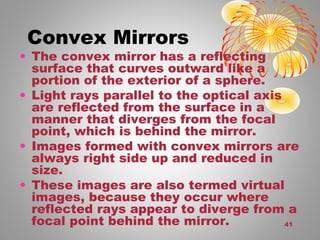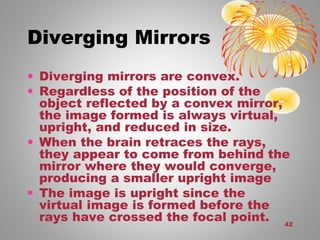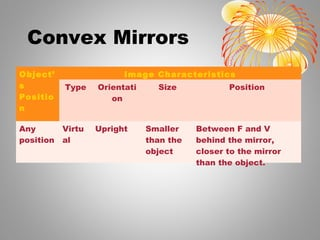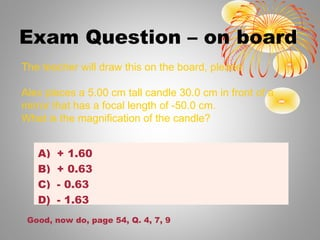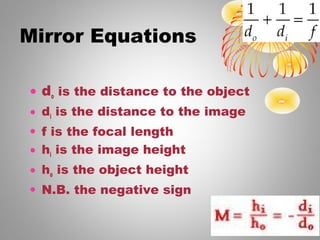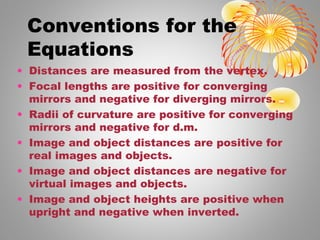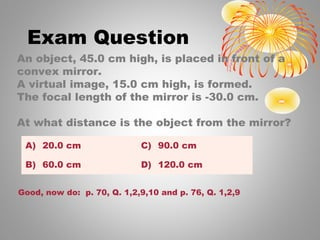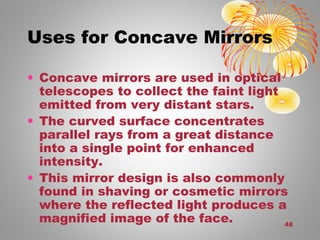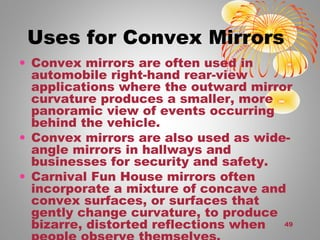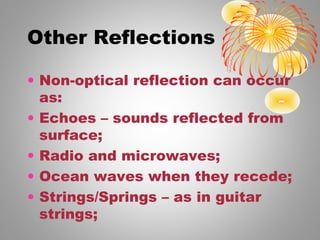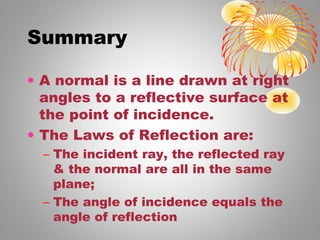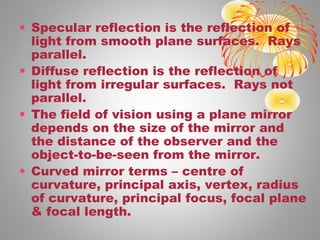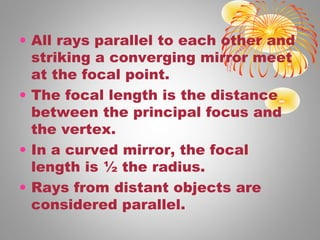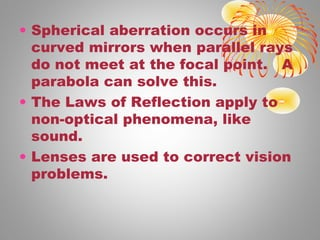1 de 54

### Physics 504 chapter 1 & 2 opticks

• 2. Alexander Pope • Nature and Nature’s laws lay hid in night; • God said, Let Newton be! • And all was light. • Epitaph intended for Sir Isaac Newton
• 3. Light • Light is an electromagnetic disturbance whose propagation allows the transportation of light energy. • Light is considered to travel as a wave AND as particle or photon. • As a wave, light has different frequencies or cycles per second (cps) • The unit of frequency is a hertz (Hz).
• 4. Transmission of light • Recti-Linear propagation: light travels in straight lines; • Ray: the path taken by light energy; • Ray diagrams: pictures that show the paths of light rays; • Diverge: light rays become farther apart; • Converge: light rays come closer together; • Parallel rays: rays that travel the same distance apart; • Speed of light: c = 3x108 m/s
• 5. Speed of light • Light travels at a definite speed (c); • c = 3x108 m/s = 300000 km/s • Distance = speed x time • Calculate the distance (km) travelled in: • 1 minute • 1 hour • 1 day • 1 year - 365.25 days – 1 lightyear
• 7. Pinhole camera • Pinhole cameras demonstrate that light travels in straight lines; • An image is formed inverted vertically and horizontally on the back of the camera • The image will be inverted, smaller and real.
• 8. Pinhole camera • Magnification = height of image height of object • M = h i/ h o • As well, M = distance to image distance to object • Or, M = d I/d O • So h i/ h o = d I/d O
• 9. QuestionA pinhole camera is used to show the image of a turtle on its screen as shown below. Which of the following is the image of the turtle that will appear on the screen of the pinhole camera? A) C) B) D)
• 10. Question Alex places a 5.00 cm tall candle 30.0 cm in front of a pinhole camera that has a length of 50.0 cm. What is the magnification of the candle? A) 1.66 B) 0.166 C) 0.63 D) 0.1
• 11. Shadow • Umbra: the area of total shadow – this area receives no light; • Penumbra: the area of partial shadow – this area receives some light; • E.g. lunar/solar eclipses
• 12. Question When only the Moon's penumbral shadow strikes Earth, we see a partial eclipse of the Sun from that region. In which region(s) on Earth do we see a partial eclipse of the Sun? A) Region A only B) Region B only C) Regions A and C D) Regions A, B and C
• 13. Activity • Page 35, Q. 2,6,7,8,9 • Page 40, Q. 1-3, 5
• 14. Summary • A luminous light source emits its own light. • A luminous light source can produce its light by incandescence, luminescence, fluorescence, or phosphorescence. • Light striking an object can be reflected, refracted, absorbed or any combo of the 3. • Other light phenomena: diffraction, diffusion, scattering, interference, dispersion. • Light travels in straight line ray paths – rectilinear propagation.
• 15. Summary • When an object creates a shadow, the area of total shadow is the umbra, the area of partial shadow is the penumbra. • For a pinhole camera, M, the magnification is given by • M = hi/ho = di/do.
• 17. Vocabulary • Reflection in a plane mirror, • Incident ray – ray coming into surface • Point of incidence – where the ray hits the surface • Reflected ray – the reflected ray • Normal – line at 90 ⁰ to point of incidence.
• 18. Vocabulary • Image Type • Virtual only appears in the eye of the beholder • Real can be put on a screen • Attitude or Orientation – upright or inverted (upside down) • Size – smaller, same, bigger
• 19. Types of Reflection • Diffuse reflection occurs when the reflected rays of light are not parallel. • The surface is usually rough and uneven. • E.g. paper, snow
• 20. Types of Reflection • Specular (regular) reflection occurs when the reflected rays of light emerge parallel from the surface. • The surface is usually smooth and polished. • E.g. mirror, glass
• 21. Rays Incident Rays Reflected Rays normal normal Angle of incidence Angle of reflection
• 22. Angle of Incidence & Reflection • The angles of incidence and reflection are measured relative to the NORMAL – • NOT THE SURFACE. • The angle of incidence equals the angle of reflection. • The incident ray, the reflected ray and the normal are all in the same plane.
• 23. Formation of Image by a Plane Mirror – Ray Diagram • A ray of light incident on a plane mirror at 90o gets reflected from the mirror along the same path. • A ray of light falling on a plane mirror at any angle gets reflected from the mirror such that the angle of incidence is equal to the angle of reflection. • P. 45, Q. 1, 3
• 24. Images formed in a Plane Mirror • A plane mirror is a flat mirror. • Images are the same attitude – upright or inverted. • Images are the same distance “behind” the mirror as the object is in front of it. • Images formed are virtual – they cannot be projected on a screen. • A line joining the image and object is perpendicular to the plane mirror.
• 25. QuestionQuestion An object is placed in front of a plane mirror. Which of the following diagrams correctly represents the image formed in the plane mirror? A) C) B) D)
• 26. QuestionQuestion An object is placed in front of a plane mirror. Which statement correctly describes the characteristics of the image? A) The image is real, upright, smaller than the object and located in front of the mirror. B) The image is virtual, inverted, larger than the object and located behind the mirror. C) The image is real, inverted, the same size as the object and located in front of the mirror. D) The image is virtual, upright, the same size as the object and located behind the mirror.
• 27. Exercise • Using a light beam, ruler and plane mirror, draw the incident, normal and reflected ray coming off the mirror. • Compare the angles. • __________________________________
• 28. Field of Vision • The field of vision from a mirror is determined by the width of the mirror, the distance of the observer to the mirror and its curvature. • In stores curved mirrors are used to give a wider field of vision.
• 29. FIELD OF VISION normal normal Field of Vision Observer Θr Θi Θr Θi Plane mirror
• 30. Field of Vision • Our view in a mirror depends on how we position ourselves in front or to the side of the mirror. • The field of vision can be determined through ray diagrams: • a. Draw two normals, one at either end of the mirror, • b. Draw an incident ray from your eye to each normal. • c. Draw a reflected ray from each normal. • d. Remember the laws of reflection when drawing both sets of rays (i = r) • Make a diagram – ask teacher.
• 31. Activity: Determine FieldActivity: Determine Field of Vision:of Vision: for a Plane Mirrorfor a Plane Mirror ________MirrorMirror__________ OO
• 32. Activity: Determine FieldActivity: Determine Field of Vision:of Vision: For a Curved MirrorFor a Curved Mirror OO
• 33. QuestionQuestion An observer is standing in front of a reflective window in which he can see the images of some shrubs. Based on the above diagram, the images of which shrubs can be seen by the observer? A) III only B) II and III only C) III and IV only D) I, II, III and IV Good, now answer: p. 47, Q. 1-3
• 35. 35 The Inside of A Spoon • Why is it when you look into a soup spoon that your image turns upside down sometimes? • The inside of a spoon is a concave mirror. • If the inside of the spoon is held close to the eye, a magnified upright view of the eye will be seen (in this case the eye is closer than the focal point of the mirror). • If the spoon is moved farther away, a smaller upside-down view of the whole face will be seen.
• 36. 36 Concave Mirrors • The concave mirror has a reflection surface that curves inward, like a portion of the interior of a sphere. • When light rays that are parallel to the principal or optical axis reflect from the surface of a concave mirror, they converge on the focal point (black dot) in front of the mirror.
• 37. 37 Converging Mirror • Concave mirrors bring light rays to a focus and are called converging mirrors.
• 38. 38 Rules for Con/Di-Verging Mirrors • A ray that is parallel to the principal axis is reflected through the principal focus – real or virtual. • A ray passing through the principal focus is reflected parallel to the principal axis. • A ray passing through the centre of curvature is reflected back along the same path. • The centre of curvature or radius is twice the focal length.
• 39. QuestionQuestion Look at the diagram below. A 12 cm tall object is placed in front of a concave mirror. The focal length is 30 cm. The object is located 70 cm from the top of the mirror. What will be the height of the image reflected by the mirror? A) 5.1 cm B) 9.0 cm C) 16 cm D) 28 cm
• 40. Concave Mirrors Object’ s Positio n Image Characteristics Type Orientati on Size Position At ∞ Real Point Image At F Beyond C Real Inverted Smaller Between F and C At C Real Inverted Same size At C Between C and F Real Inverted Bigger Beyond C At F No Image Between F and V Virtual Upright Larger than the object Between F and V behind the mirror, farther from the mirror than the object.
• 41. 41 Convex Mirrors • The convex mirror has a reflecting surface that curves outward like a portion of the exterior of a sphere. • Light rays parallel to the optical axis are reflected from the surface in a manner that diverges from the focal point, which is behind the mirror. • Images formed with convex mirrors are always right side up and reduced in size. • These images are also termed virtual images, because they occur where reflected rays appear to diverge from a focal point behind the mirror.
• 42. 42 Diverging Mirrors • Diverging mirrors are convex. • Regardless of the position of the object reflected by a convex mirror, the image formed is always virtual, upright, and reduced in size. • When the brain retraces the rays, they appear to come from behind the mirror where they would converge, producing a smaller upright image • The image is upright since the virtual image is formed before the rays have crossed the focal point.
• 43. Convex Mirrors Object’ s Positio n Image Characteristics Type Orientati on Size Position Any position Virtu al Upright Smaller than the object Between F and V behind the mirror, closer to the mirror than the object.
• 44. Exam Question – on board A) + 1.60 B) + 0.63 C) - 0.63 D) - 1.63 The teacher will draw this on the board, please. Alex places a 5.00 cm tall candle 30.0 cm in front of a mirror that has a focal length of ‑50.0 cm. What is the magnification of the candle? Good, now do, page 54, Q. 4, 7, 9
• 45. Mirror Equations • do is the distance to the object • di is the distance to the image • f is the focal length • hi is the image height • ho is the object height • N.B. the negative sign
• 46. Conventions for the Equations • Distances are measured from the vertex. • Focal lengths are positive for converging mirrors and negative for diverging mirrors. • Radii of curvature are positive for converging mirrors and negative for d.m. • Image and object distances are positive for real images and objects. • Image and object distances are negative for virtual images and objects. • Image and object heights are positive when upright and negative when inverted.
• 47. Exam Question A) 20.0 cm C) 90.0 cm B) 60.0 cm D) 120.0 cm An object, 45.0 cm high, is placed in front of a convex mirror. A virtual image, 15.0 cm high, is formed. The focal length of the mirror is -30.0 cm. At what distance is the object from the mirror? Good, now do: p. 70, Q. 1,2,9,10 and p. 76, Q. 1,2,9
• 48. 48 Uses for Concave Mirrors • Concave mirrors are used in optical telescopes to collect the faint light emitted from very distant stars. • The curved surface concentrates parallel rays from a great distance into a single point for enhanced intensity. • This mirror design is also commonly found in shaving or cosmetic mirrors where the reflected light produces a magnified image of the face.
• 49. 49 Uses for Convex Mirrors • Convex mirrors are often used in automobile right-hand rear-view applications where the outward mirror curvature produces a smaller, more panoramic view of events occurring behind the vehicle. • Convex mirrors are also used as wide- angle mirrors in hallways and businesses for security and safety. • Carnival Fun House mirrors often incorporate a mixture of concave and convex surfaces, or surfaces that gently change curvature, to produce bizarre, distorted reflections when
• 50. Other Reflections • Non-optical reflection can occur as: • Echoes – sounds reflected from surface; • Radio and microwaves; • Ocean waves when they recede; • Strings/Springs – as in guitar strings;
• 51. Summary • A normal is a line drawn at right angles to a reflective surface at the point of incidence. • The Laws of Reflection are: – The incident ray, the reflected ray & the normal are all in the same plane; – The angle of incidence equals the angle of reflection
• 52. • Specular reflection is the reflection of light from smooth plane surfaces. Rays parallel. • Diffuse reflection is the reflection of light from irregular surfaces. Rays not parallel. • The field of vision using a plane mirror depends on the size of the mirror and the distance of the observer and the object-to-be-seen from the mirror. • Curved mirror terms – centre of curvature, principal axis, vertex, radius of curvature, principal focus, focal plane & focal length.
• 53. • All rays parallel to each other and striking a converging mirror meet at the focal point. • The focal length is the distance between the principal focus and the vertex. • In a curved mirror, the focal length is ½ the radius. • Rays from distant objects are considered parallel.
• 54. • Spherical aberration occurs in curved mirrors when parallel rays do not meet at the focal point. A parabola can solve this. • The Laws of Reflection apply to non-optical phenomena, like sound. • Lenses are used to correct vision problems.

### Notas do Editor

1. Chapter Slide
2. Pinhole camera